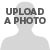# MapInfo Pro

View Only

## Square ended buffer of line?#### Nick Lawrence19 days ago#### James Nolet19 days ago#### Nick Lawrence19 days ago#### James Nolet18 days agoBest Answer#### Nick Lawrence18 days ago• #### 1.  Square ended buffer of line?

Posted 19 days ago
Hello all,

I would like to buffer polylines with a square ended buffer.
Instead of the normal semi-circle end, I would like a square end that is flat against the end of the polyline.
Is there a way to do this? Either in MapInfo or in MapBasic?

------------------------------
Nick Lawrence
TMR-Policy and Planning
Brisbane QLD
------------------------------

• #### 2.  RE: Square ended buffer of line?

Posted 19 days ago
Hi Nick,

Try Create Parallel from the Buffer drop-down in the Spatial menu.  It seems to do the trick.  You can create parallel lines on either side of your existing lines, close them off and even create them as regions.

------------------------------
James Nolet
Dooley Mitchell & Morrison Pty Ltd
Mentone, VIC, Australia
------------------------------

• #### 3.  RE: Square ended buffer of line?

Posted 19 days ago
Thanks James, that is exactly the result I want.
Is there a corresponding mapbasic command?
I suspect that this function is a part of MapCAD. Is there a way for MapBasic to access MapCAD functions?

------------------------------
Nick Lawrence
TMR-Policy and Planning
Brisbane QLD
------------------------------

• #### 4.  RE: Square ended buffer of line? Best Answer

Posted 18 days ago
Hi Nick,

Yes, Create Parallel comes with the MapCAD tool.  I forgot about that, as I always have it loaded and end up taking its functionality for granted.

Checking MapBasic Help I can see that you can call the MapCAD functions.  For example, if you select a single line and execute the following you will convert the selected line to a buffer region:

Dim oPolyline as Object
oPolyline = Selection.obj
Update Selection Set obj = Exec("MapCAD.mbx","CreateParallel", oPolyline, 0.2, 3, "T", "T")

(where 0.2 is the buffer distance, "3" means create parallel lines on either side, and the "T"s mean close the lines and form a region).

So using a loop you could create a buffer for each line in your table one record at a time.  You will need MapCAD to be running of course.

------------------------------
James Nolet
Dooley Mitchell & Morrison Pty Ltd
Mentone, VIC, Australia
------------------------------

• #### 5.  RE: Square ended buffer of line?

Posted 18 days ago
Thank you James

------------------------------
Nick Lawrence
TMR-Policy and Planning
Brisbane QLD
------------------------------

• #### 6.  RE: Square ended buffer of line?

Posted 17 days ago
Found a custom function you could try out in MapBasic.  Have not tried it myself in an application, but it came from a shared library on github.

' Creates a square buffer around an object
' Parameters:
' oInput: input object
' fDist: distance to buffer
' Returns:
' object buffered by a square buffer (approximate only)
Declare Function SquareBuffer(ByVal oInput as Object, ByVal fDist as Float) as Object

Function SquareBuffer( ByVal oInput As Object, ByVal fDist As Float ) As Object
Dim fX1, fY1, fX2, fY2, fXRange, fYRange, fAngle, fDeltaX, fDeltaY As Float
Dim iQuadrant As Integer
Dim fBuffer(8) As Float
Dim oTemp As Object

' Get line start and end points
fX1 = ObjectGeography( oInput, OBJ_GEO_LINEBEGX )
fY1 = ObjectGeography( oInput, OBJ_GEO_LINEBEGY )
fX2 = ObjectGeography( oInput, OBJ_GEO_LINEENDX )
fY2 = ObjectGeography( oInput, OBJ_GEO_LINEENDY )

' Calculate range
fXRange = Abs( fX1 - fX2 )
fYRange = Abs( fY1 - fY2 )

' Calculate angle of line
If ( fYRange = 0 ) Then
fAngle = 90
Else
fAngle = Atn( fXRange / fYRange ) * RAD_2_DEG
End If

fDeltaX = Cos( fAngle * DEG_2_RAD ) * fDist
fDeltaY = Sin( fAngle * DEG_2_RAD ) * fDist

If ( fX2 - fX1 ) > 0 Then
If ( fY2 - fY1 ) > 0 Then
Else
End If
ElseIf ( fY1 - fY2 ) > 0 Then
Else
End If

' Calculate buffer extents based on quadrant
Case 1
fBuffer(1) = fX1 - fDeltaX
fBuffer(2) = fY1 + fDeltaY
fBuffer(3) = fX1 + fDeltaX
fBuffer(4) = fY1 - fDeltaY
fBuffer(5) = fX2 + fDeltaX
fBuffer(6) = fY2 - fDeltaY
fBuffer(7) = fX2 - fDeltaX
fBuffer(8) = fY2 + fDeltaY
Case 2
fBuffer(1) = fX1 + fDeltaX
fBuffer(2) = fY1 + fDeltaY
fBuffer(3) = fX1 - fDeltaX
fBuffer(4) = fY1 - fDeltaY
fBuffer(5) = fX2 - fDeltaX
fBuffer(6) = fY2 - fDeltaY
fBuffer(7) = fX2 + fDeltaX
fBuffer(8) = fY2 + fDeltaY
Case 3
fBuffer(1) = fX1 + fDeltaX
fBuffer(2) = fY1 - fDeltaY
fBuffer(3) = fX1 - fDeltaX
fBuffer(4) = fY1 + fDeltaY
fBuffer(5) = fX2 - fDeltaX
fBuffer(6) = fY2 + fDeltaY
fBuffer(7) = fX2 + fDeltaX
fBuffer(8) = fY2 - fDeltaY
Case 4
fBuffer(1) = fX1 - fDeltaX
fBuffer(2) = fY1 - fDeltaY
fBuffer(3) = fX1 + fDeltaX
fBuffer(4) = fY1 + fDeltaY
fBuffer(5) = fX2 + fDeltaX
fBuffer(6) = fY2 + fDeltaY
fBuffer(7) = fX2 - fDeltaX
fBuffer(8) = fY2 - fDeltaY
End Case

' Make a new region object matching the extents
Create Region Into Variable oTemp 1
5 ( fBuffer(1), fBuffer(2))( fBuffer(3), fBuffer(4))( fBuffer(5), fBuffer(6))( fBuffer(7), fBuffer(8))( fBuffer(1), fBuffer(2))

SquareBuffer = oTemp

End Function

Regards,
-Bill

------------------------------
Bill Wemple
Principal Software Engineer, Quality Management
Precisely, Inc
precisely.com
------------------------------